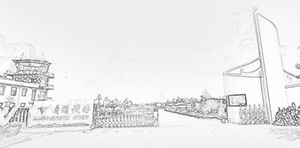﻿ 吴东伦-理学院1. 项目名称：Hamilton 系统同宿轨与双曲轨的研究

项目来源：国家自然基金数学天元基金(11626198)

起止时间：2017.01-2017.12

2. 项目名称：渐近周期Hamilton 系统同宿轨的研究

项目来源：国家自然基金青年基金(11801472)

起止时间：2019.01-2021.12

主研的国家级项目：

3. 项目名称：多体问题的解的研究

项目来源：国家自然科学基金面上项目(11071175)

起止时间：2011.01-2013.12

4. 项目名称：概周期KAM理论及其应用

项目来源：自然科学青年基金(11301358)

起止时间：2014.01-2016.12

1. D.-L. Wu, C.-L. Tang, X.-P. Wu, Homoclinic orbits for a class of second-order Hamiltonian systems with concave-convex nonlinearities, Electronic Journal of Qualitative Theory of Differential Equations, 6 (2018)

2. D.-L. Wu, C. Li, P.F. Yuan, Multiplicity solutions for a class of fractional Hamiltonian systems with concave-convex potentials, Mediterranean Journal of Mathematics, 15 (2018)

3. D.-L. Wu, X. Yu, New homoclinic orbits for Hamiltonian systems with asymptotically quadratic growth at infinity, Qual. Theory Dyn. Syst. 19 (2020).

4. D.-L. Wu, F. Li, Solutions for fourth-order Kirchhoff type elliptic equations involving concave-convex nonlinearities in RN, Comput. Math. Appl. 79(2) (2020)

5. D.-L. Wu, H.X. Lin, Multiple solutions for superlinear Klein–Gordon–Maxwell equations. Mathematische Nachrichten. 2020

6. D.-L. Wu，Existence and Multiplicity of Solutions for Sublinear Schrödinger Equations with Coercive Potentials, Mathematical Problems in Engineering, (2019).

7. D.-L. Wu, C.-L. Tang, X.-P. Wu, Existence and nonuniqueness of homoclinic solutions for second-order Hamiltonian systems with mixed nonlinearities, Communications on Pure and Applied Analysis, 15 (2016)

8. D.-L. Wu, C. Li, P.F. Yuan, Periodic solutions for a class of second-order Hamiltonian systems of prescribed energy, Electronic Journal of Qualitative Theory of Differential Equations, 2015 (2015)

9. D.-L. Wu，C.-L. T.，X.-P. Wu，Subharmonic and homoclinic solutions for second order Hamiltonian systems with new superquadratic conditions， Chaos, Solitons & Fractals, 73 (2015)

10. D.-L. Wu, S.Q. Zhang，New hyperbolic orbits for a class of singular Hamiltonian systems, Boundary Value Problems, 2015:86 (2015) .

11. D.-L. Wu, S.Q. Zhang， Homoclinic Orbits to Infinity for Second Order Hamiltonian Systems with Fixed Energy, Electronic Journal of Differential Equations, 2015 (2015)

12. D.-L. Wu，X.-P. Wu，C.-L. Tang， Homoclinic solutions for second order Hamiltonian systems with small forcing terms，Bulletin of the Belgian Mathematical Society-Simon Stevin, 19 (2012)

13. D.-L. Wu，X.-P. Wu，C.-L. Tang，Homoclinic solutions for a class of nonperiodic and noneven second-order Hamiltonian systems. Journal of Mathematical Analysis and Applications, 367 (2010)

14. Qin Zheng, D.-L. Wu, Multiple Solutions for Schrödinger Equations Involving Concave-Convex Nonlinearities Without (AR)-Type Condition, Bull. Malays. Math. Sci. Soc. (2021)

15. C. Li, R. P. Agarwal and D.-L. Wu, Existence and multiplicity of solutions for a class of superlinear elliptic systems, Advances in Nonlinear Analysis, 2018.

16. C. Li, Z.-Q. Ou, D.-L. Wu, On the existence of minimal periodic solutions for a class of second-order Hamiltonian systems，Applied Mathematics Letters, 43 (2015), 44-48.

17. C.H. Deng, D.-L. Wu, Multiple homoclinic solutions for a class of nonhomogeneous Hamiltonian systems, Boundary Value Problems, 2018:56 (2018).

D.-L. Wu，X.-P. Wu, C.-L. Tang, Existence of homoclinic solutions for a class of second-order Hamiltonian system with c